ctf

We were given an apk (Login.apk). using dex2jar + jd we can see decompiled code of the apk.

In MainActivity class we have following onCreate method: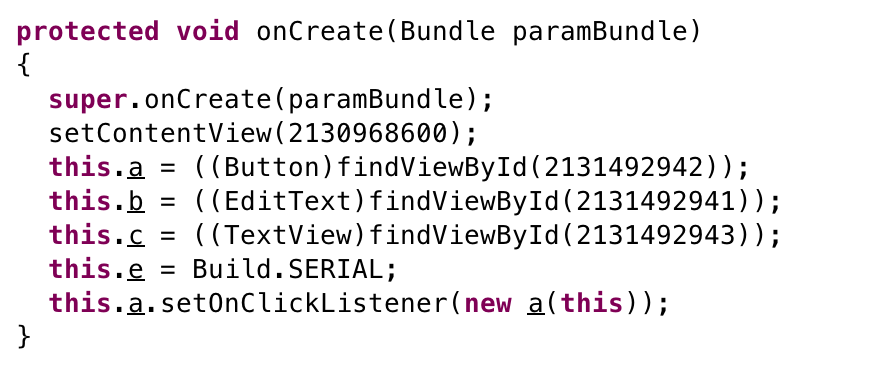this.a is a Button and last line set a listener a class on it: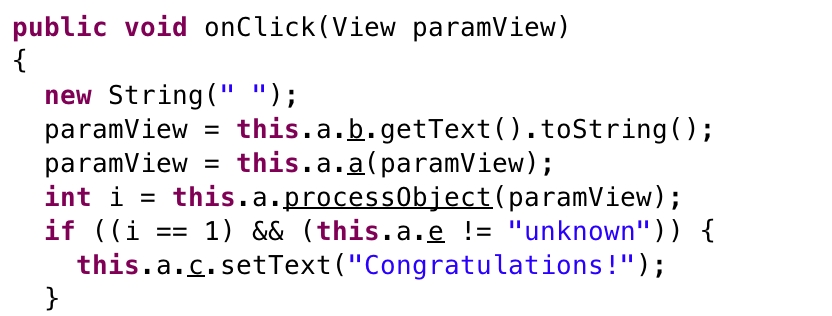We are interested in (i==1 & (this.a.e != “unknown”)). the second part is not necessary since it is initialized with Build.SERIAL. So we are dealing with (i==1):

paramView = this.a.a(paramView);

this.a is MainActivity class and this.a.a is: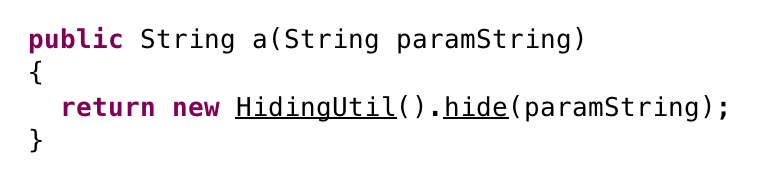According to HidingUtil we have following code: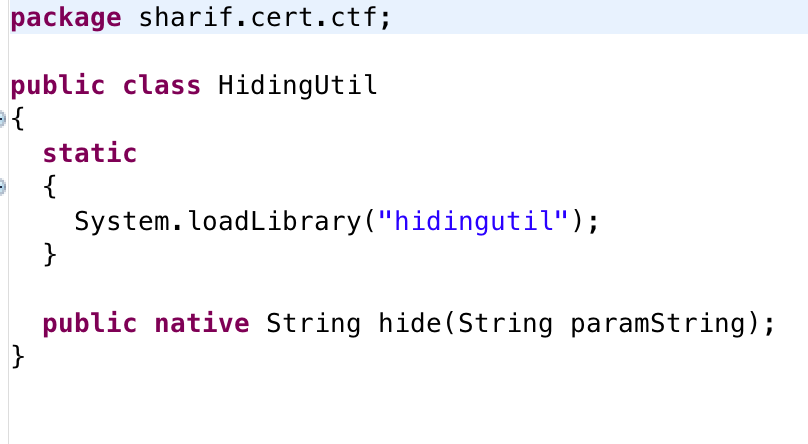So hidingutil library is important to us. besides we have following line:

int i = this.a.processObject(paramView);

and we can see processObject is also a hidingUtil library function.

Let’s disassemble libhidingutil.so:

processObject disassembly: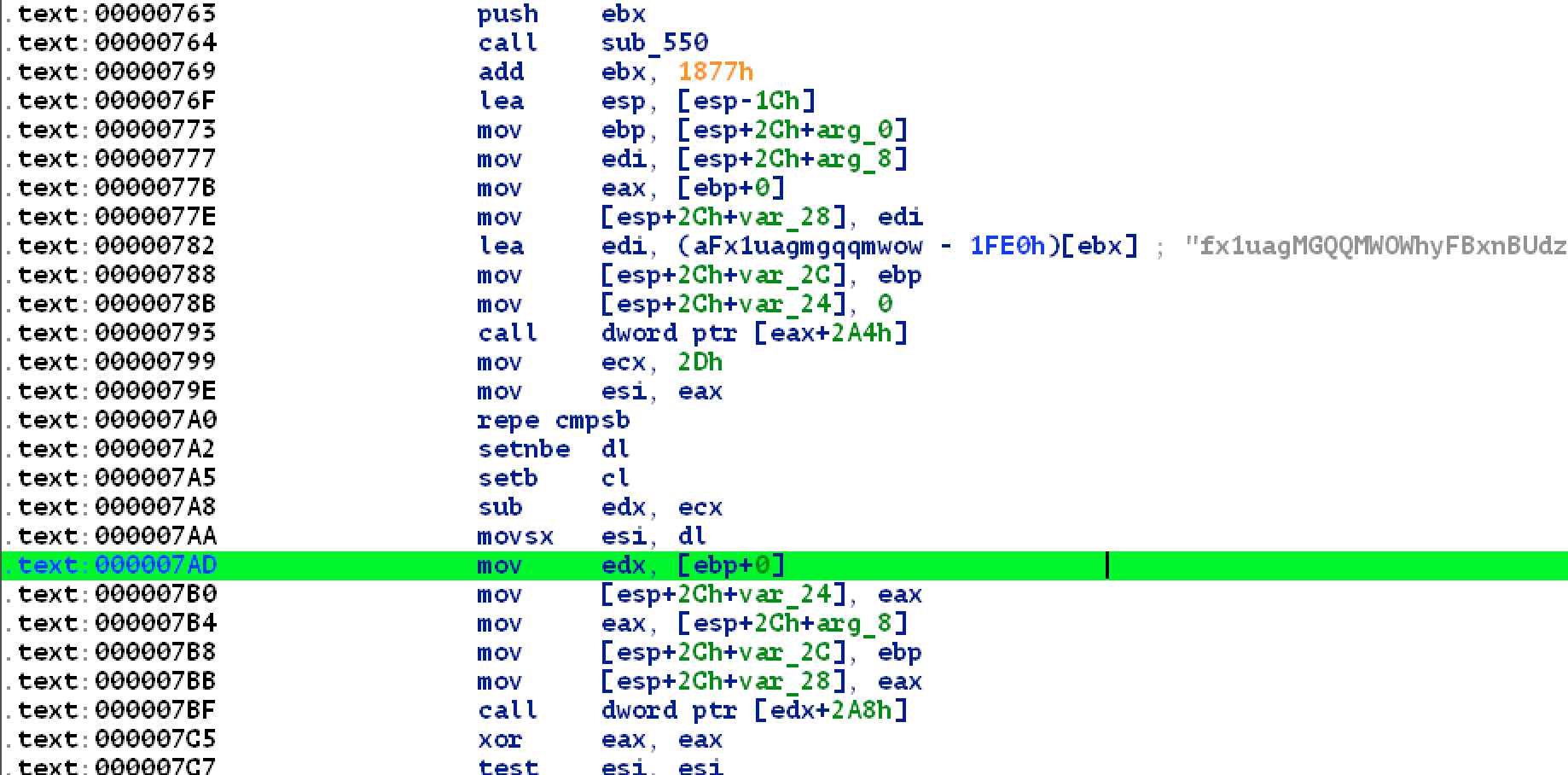and its psuedo code:

int Java_sharif_cert_ctf_MainActivity_processObject(char *input)
{
char *out = "fx1uagMGQQMWOWhyFBxnBUdzN35NPWYHUBQHRmozeEY=";
int len = 45;
char *ptr = input;
int check = 0;
do
{
if (!len)
break;
check = *ptr++ == *out++;
len--;

} while (check);
return check == 0;
}

HidingUtil_hide disassembly:after cleaning function and removing unnecessary parts we have following pseudo code:

void value_with_key(inb, outb)
{
char *aMy_s3cr3t_p_W0 = "My_S3cr3t_P@\$\$W0rD"; // .rodata
char l;
int idx = 0;
for (l=inb; l; l = inb[idx])
{
out[idx] = aMy_s3cr3t_p_W0[idx % 0x13] ^ l;
idx++;
}
return;
}

char *Java_sharif_cert_ctf_HidingUtil_hide(char *input)
{
char out;
value_with_key(input, out);
char *rv = Base64Encode(out);
return rv;
}

So what is we should solve is:

Java_sharif_cert_ctf_MainActivity_processObject(Java_sharif_cert_ctf_HidingUtil_hide(“flag”)) == 1;

And our solver code:

#!/usr/bin/python

a = "fx1uagMGQQMWOWhyFBxnBUdzN35NPWYHUBQHRmozeEY="
pw = "My_S3cr3t_P@\$\$W0rD\x00"
print len(pw)
a = a.decode("base64")

b = ""
i = 0
for c in a:
b += chr(0xff & (ord(c) ^ ord(pw[i % 0x13])))
i += 1

print "flag:", b